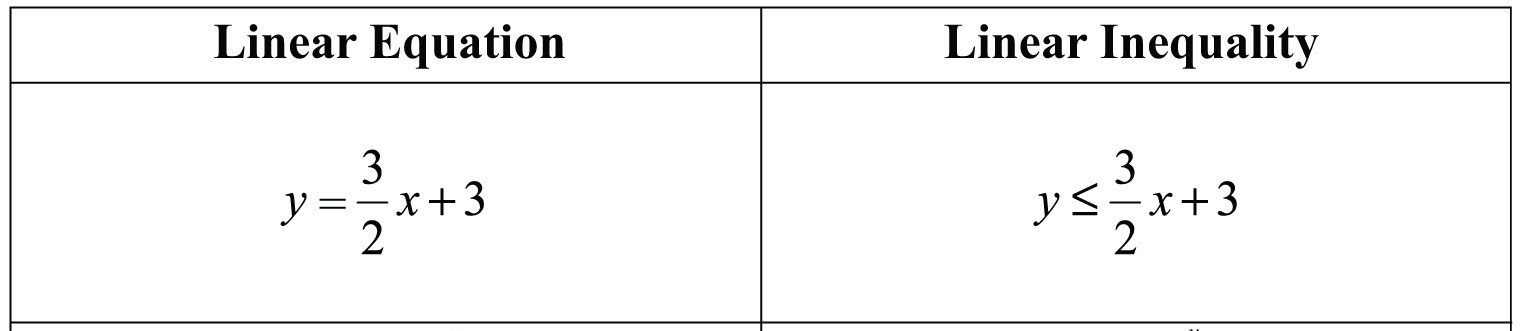# Linear inequalities in one variable/graphical representation

Welcome to Class !!

In today’s Mathematics class, We will be discussing Linear Inequalities in one variable / graphical representation. We hope you enjoy the class!

CONTENT

• Solution of inequalities
• Multiplication and division of negative numbers
• Word problem involving inequalities#### Solution of inequalities

Inequalities are solved in the same way as a simple equation

For Example x = 23 is equation

While x < 3 is an inequality.Worked Examples

1. Solve the inequality and show the Solution on a graph.
1. x + 4 < 6
2. 6  <  2x – 1
3. 3x – 3 > 7

Solution

(1) x + 4 < 6

x < 6 – 4

x  < 2

x

-2       -1      0      1      2       3

(2) 6  2x – 14

<   2x

3 ½  <  x

X  >  3 ½

x   >  3 ½

3 ½

(3) 3x – 3 > 7

3x > 7 + 3

3x > 10

x  > 10/3

x   > 3

x   >  3 ½

-2    -1  0   1   2   3   3 1/3    4     5    6   7

EVALUATION

1. Solve the following inequalities and show the Solution on a graph:
1. 5x -2   >  8
2. 4x – 2 > 19
3. 3  <  3x   X 5
4. x  +  4   >   10

#### MULTIPLICATION AND DIVISION BY NEGATIVE NUMBER

When solving an inequality involving negative numbers, the inequality sign must be reversed. For Example if

-2x > 10 is true then on division throughout by -2.

X <- 5 will be true

Worked Examples

1. Solve 5 – x > 3
2. Solve 19 >  4 -5x
3. Solve 3 – 2x < 8

Solution

(1) 5 – x > 3

-x > 3 – 5

-x > -2

Dividing through by ( -1)

X < 2

(2) 19 >  4 – 5x

19 – 4  >  – 5x

15  >  – 5x

Dividing through by -5

-3  <  -5x

x  >  – 3

(3) 3 – 2x < 8

-2x < 8 – 3

Evaluation

Solve the following inequalities

1. -2x + 5 > 16
2. 10 – 3x <  – 11
3. 2r  6r + 6
4. 9 <  3 – 4t

Show your Solution on a number line

New General Mathematics UBE Edition, Chapter 22, Nos 1- 2pgs 213-215

Essential Mathematics by A. JS. Oluwasanmi Chapter 23 pgs 40 – 243

#### WORD PROBLEMS INVOLVING INEQUALITIES

Worked Example

1. A triangle has sides x cm, ( x + 4) cm and 11cm, where x is a whole number of cm if the perimeter of the triangle is less than 32cm. find the possible values of x.

Solution

Perimeter of triangle = x + ( x + 4) + 11

But perimeter < 32cm

x+ 4 +11< 32

2x < 32 – 15

2x < 17

x< 17/2

x < 8 ½

Also in any triangle, the sum of the length of any two sides must be greater than the length of the third side.

Thus,

X + (x + 4) > 11

2x + 4 > 11

2x > 7

x > 7/2

x > 3 ½

Thus

x< 8 ½ and x > 3 ½ .  but x is a whole number of cm therefore, the possible values of x are 4,5,6,7 or 8.

GENERAL EVALUATION

1. If 9 is added to a number x, the result is greater than 17. Find the values of x
2. Three times a certain number is not greater than 54. Find the range of values of the number.

REVISION QUESTION

1. If 8 is subtracted from a number, the result is at most 15. Find the range of values of the number
2. If x  is subtracted from 5, and the result is greater than 15. Find the range of values of x.

WEEKEND ASSIGNMENT

1. Find which symbol > or < goes in the box to make the statement 9 + 8 10 true.
• A) < B.) >     C.) none of the above
2. Write the inequality of the statement ‘The cost of meal Nx was over N5.  A) x > N5  (B) x > N5   (C.) x < N 5
3. Solve 5x – 7 > 9    (A.) x > 32.5   (B.)  x > 3 1/5    (C.)  x , 5 2/3
4. Solve x -2 < 3    (A.)  x < 5   (B.)  x > 5    (C.) x  > 5
5. If 7.3 is subtracted from y, the result is less than 3.4. find the value of y.
• (A.) y < 10.7   (B.) y > 10.7   (C.) y <  10.7

THEORY

1. A rectangle is 8cm long and b cm branch find the value of b if the perimeter of the rectangle is not greater than 50cm and not less than 18cm
2. The sides of a rectangle are x cm (x+ 3cm) and 10cm. if x cm is a whole number, if the perimeter is less than 30cm, find the possible value of x.

Hint: The sum of the length of any two sides of a triangle must be greater than the length of the third side.

We have come to the end of this class. We do hope you enjoyed the class?

Should you have any further question, feel free to ask in the comment section below and trust us to respond as soon as possible.

In our next class, we will be talking about Graph of Linear Equations in two variables. We are very much eager to meet you there.

Are you a Parent? Share your quick opinion and win free 2-month Premium Subscription

Don`t copy text!